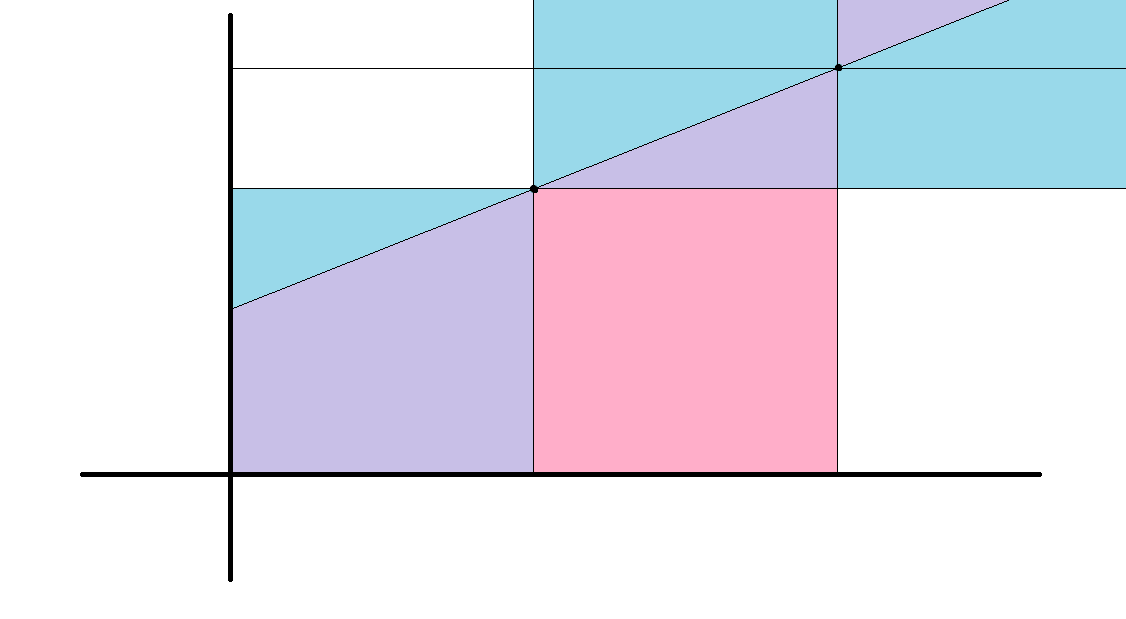# AGC选㗅

## fuck

Posted by ShanLunjiaJian's Blog on August 31, 2021

AGC001B : 你发现这是一个类似于辗转相除的过程，所以就除就行了。存在直接用gcd算答案的方法，不过我不会推。

AGC001C : 看起来好像有点好玩。首先直径上的点是一定要被删的，但是我们删哪一边呢?

AGC001D : 考虑那个回文的条件说的是什么。我不知道（

AGC001E : 来好玩的了。数数题，虽然不够数数。

$\sum_{k=0}^{v}\left(\sum_{i=1}^n\binom{a_i+b_i}{a_i+k}\right)\left(\sum_{j=1}^n\binom{a_j+b_j}{a_j-k}\right)$

$A(z)=\sum_{i=1}^nz^{v-a_i}(1+z)^{a_i+b_i}$

。注意到乘$$(1+z)^{a_i+b_i}$$是容易的，所以我们枚举$$a_i+b_i$$，此时对应的所有$$z^{v-a_i}$$加起来得到一个多项式(这也是为什么我们不枚举$$v-a_i$$，因为$$(1+z)^k$$们加起来得到的多项式不容易快速计算)，可以爆力求出它在$$0,...,2v$$的点值，然后爆力卷上$$(1+z)^{a_i+b_i}$$加入答案即可。

AGC001F : 好复杂。

AGC002C : 想了五分钟，发现时间倒流就做完了。

AGC002D : 二分一波，然后就变成可以走到Kru重构树上的两棵子树，这个可以倍增搞掉。可以走到的点数就看两棵子树是不交还是包含来算即可。

AGC002E : 注意题意是吃最后一个糖的人输了。不过实际上没啥区别（

• 一个位于第二层点的胜负只和它到右/上边界距离的奇偶性相关

• 一个位于第二层的点的胜负和它左下方一条线上所有点的胜负相同

。第二个的证明是容易的，假如它是先手必败的，那么它左边和下边是先手必胜的，那么它左下就是先手必败的。假如它是先手必胜的，意味着它左边或者上边是先手必败的，那么这个先手必败的左下方都是先手必败，所以这个先手必胜左下方也都是先手必胜。

AGC002F : 真正的AGC数数题，终于见面了（

• 填一个白球，这会使得……草?你发现这需要记一车东西，所以我们并不能这么决策。考虑一些别的决策方法。

AGC003B : 容易想到网络流，但是这个题的限制并不能用网络流表述。

AGC003C : 草?

AGC003D : 如果分解了就好说了，我们只需要次数膜$$3$$分成若干等价类，然后$$3$$进制$$\mathrm{xor}$$意义下相反的等价类不能共存，取大小更大的即可。

AGC003E : 可 持 久 化 平 衡 树

AGC003F : 考虑两个一级分形拼起来会发生什么事情。

AGC004A : 尝试从每一维中间切开。

AGC004B : 考虑我们可以在shift的时候在不同的时刻加入那些要shift不同次数的，所以枚举shift多少次，问题变成若干个区间$$\min$$加起来。单调队列就做完了。

AGC004C : 正叔给了一个不依赖边界为空性质的，但是那个太复杂，考虑我们奇数行在第一个涂黑，偶数行在第二个涂黑，第一个涂黑左边，第二个涂黑右边，这样就赢麻了。

AGC004D : 内向基环树。

AGC004E : 看起来比较复杂/jk

AGC004F : 看起来非常简单，但完全不简单/jk

$\vert a_1-x\vert+\vert a_2+a_1-x\vert+\vert a_3+a_2+a_1-x\vert+...+\vert a_k+...+a_1\vert$

AGC005A : 中文题面搞什么（

AGC005B : 考虑每个数的贡献，是一个矩形，单调栈即可。

AGC005C : 考虑最大的一定是直径端点，这个值是直径长度，最小的一定是center(只有一个)或者center所在边的端点(有两个)。

AGC005E : 感觉思维难度远高于F/jy

AGC005F : 不得不想到法法塔（

$ans_k=\sum_u\left(2^n-\sum_{u\text{的子树}i}\binom{s_i}{k}\right)$

\begin{aligned} ans_k&=n2^n-\sum_{\text{任意点的子树}i}\binom{s_i}{k}\\ \sum_{\text{任意点的子树}i}\binom{s_i}{k}&=\sum_{\text{任意点的子树}i}\frac{s_i!}{k!(s_i-k)!} \end{aligned}

$\sum_ic_i\frac{i!}{k!(i-k)!}$

AGC006B : 看到中位数，想到转01，于是问题变成有若干-1,0,1，要求最后剩下一个0。

AGC006C : 非常经典。对称点是线性的，根据期望的线性性，我们可以把它拆开，于是就变成一类cf上做奇怪操作的题，差分一下就做完了。

AGC006D : 经典再现。二分转01，然后连续段会一步一步侵蚀交替段，看哪个连续段离的最近即可。这个比那个经典题要弱?

AGC006E : 看起来好鬼。

AGC006F : 看起来更鬼了。

AGC007A : 挑 战 哈 密 顿

AGC007B : 有点意思。

AGC007C : 足够厉害。

• 如果操作了第一个点和第二个点，那么$$d^\prime_1=d_3,d^\prime_2=d_4$$

• 如果操作了第二个点和第三个点，那么$$d^\prime_1=d_1+d_2+d_3,d^\prime_2=d_4$$

• 如果操作了第三个点和第四个点，那么$$d^\prime_1=d_1,d^\prime_2=d_2+d_3+d_4$$

• 如果不是上面三种情况，什么事都不会发生

，上面三个的概率都是$$\frac{1}{2n}$$，最后一个是$$\frac{2n-3}{2n}$$。综合起来，我们得到

$$d^\prime_1=\frac{(2n-1)d_1+d_2+2d_3}{2n},d^\prime_2=\frac{(2n-2)d_2+d_3+3d_4}{2n}$$，然后代进去那个等差数列就有$$a^\prime+b^\prime=\frac{(2n+7)a+(2n+2)b}{2n},2a^\prime+b^\prime=\frac{(4n+11)a+(2n+2)b}{2n}$$，解就是了。最后得到$$a^\prime=\frac{(2n+4)a}{2n},b^\prime=\frac{3a+(2n+2)b}{2n}$$。

AGC007D : 你发现这个过程肯定是，我们冲到某个点，然后回去一直走到第一个还没拿的钱，在那里等到它可以拿，然后冲回去继续往前(，这个过程中就会把这一段所有都拿走，因为一个可以拿了，走到后面就都顺着拿掉了)。

$dp(i)=\min_{j<i}\left(dp(j)+x_i-x_j+\max(2(x_i-x_{j+1}),T)\right)$

$$x_i-x_j$$这一项是没有用的，因为最后加起来总是$$x_n$$。考虑那个$$\max$$，它在一个前缀取到前一项，于是我们双指针扫那个分界点，后面用单调队列维护，前面直接记一个$$\min$$即可。复杂度是线性的。

AGC007E : 看起来就感觉很牛逼。

AGC007F : 实际上它说的是，每个字符可以控制后面的一段，后来的会覆盖先来的。好像不是很形象，怎么说呢?

AGC008A : 只需要讨论就行了。

AGC008B : 哦原来没有操作次数限制（

AGC008C : 呃看起来很简单。不过实际上确实很简单。

AGC008D : 我们先尝试塞$$i-1$$个$$i$$，如果这样的塞完了就继续塞那些已经判定完了的，等到要求的位置就塞上需要的。

AGC008E : ¿

AGC008F : 退钱，我一直以为是多次操作/jk

• 如果这个点是好点，那么下界是$$0$$，上界是各$$f$$的次大值，因为超过这个值之后就可以往最大的子树平移

• 如果这个点不是好点，那么上界同上，下界是各有好点子树$$f$$的最小值，因为我们需要包含一个好点作为中心，就必须选完它的子树，这和边作为最小中心的情况类似

AGC009A : 前缀修改考虑前缀和，相当于单点$$+1$$，最后要求$$a_i,a_{i-1}$$膜$$b_i$$相同。还是不可做¿

AGC009B : 考虑从$$i$$连到$$a_i$$，那么问题变成要把这棵树三度化，每个点建一堆虚点串成一串挂儿子，希望深度最小。按照子树高度，先干低的再干高的即可。

AGC009C : 考虑排序之后dp，设$$dp(i,0/1)$$表示考虑前$$i$$个，这一个选入集合A/B，上一个选入另一个的方案数。

$dp(i,0)=\sum_{j<i,w(j,i-1)\geq a}dp(j,1)$

$$1$$是对称的，其中$$w(i,j)$$表示$$i$$到$$j$$的最小邻项差。发现满足$$w(j,i-1)\geq a$$的是一个后缀，于是我们用前缀和维护，遇到差$$\leq a$$的就重置即可。复杂度是线性。

AGC009D : 考虑这个过程是说建立一棵重构树，我们考虑最后放的那个点，称它的级是树的Uninity，它会把树分成若干连通块，这类似点分治的过程，实际上我们就是要求高度最小的点分树。

AGC009E : suxxsfe认为的牛逼题/se

AGC010A : 先把所有数膜$$2$$。

AGC010B : ¿

AGC010C : ¿

AGC010D : ¿

AGC010E : grass，我以为是只换一次/jy

AGC010F : 硬想题。

AGC011A : 摆 渡 车

AGC011B : 考虑这就是说有多少可以冲到最后。注意到每次吸收会翻倍，所以只需要吸收$$\log$$次就可以干翻所有别的，所以就爆力判能不能吸收到1e9以上即可。用set维护。

AGC011C : ¿

AGC011D : 画一画你可以发现，我们把关着的记为1，开着的记为0，那么如果左边是1，球给左边取反之后就溜了；如果左边不是1，效果是把所有数取反，然后循环左移一位。

AGC011E : 显然我们每次可以缩减一个极长递增前缀。然后考虑尽可能多选一点，也就是后面选上9999999，然后依此模拟，不过这是AGC E，所以不是很行。

$n=\sum_i \frac{(10^i-1)c_i}{9}$

$9n+9k=\sum_i 10^ic_i$

AGC011F : 题意比较鬼。

$[sx_{p-1}+pre_{p-1},sx_{p-1}+pre_p]\cap[sy_p+suf_{p+1},sy_p+suf_p]=\varnothing$

\begin{aligned} sy_p&+suf_{p+1}&\notin&[sx_{p-1}+pre_{p-1}&,&sx_{p-1}+pre_p]\\ sy_p&+suf_p&\notin&[sx_{p-1}+pre_{p-1}&,&sx_{p-1}+pre_p]\\ sx_{p-1}&+pre_{p-1}&\notin&[sy_p+suf_{p+1}&,&sy_p+suf_p]\\ sx_{p-1}&+pre_p&\notin&[sy_p+suf_{p+1}&,&sy_p+suf_p] \end{aligned}

\begin{aligned} sy_p-sx_{p-1}&\notin&[pre_{p-1}-suf_{p+1}&,&pre_p-suf_{p+1}]\\ sy_p-sx_{p-1}&\notin&[pre_{p-1}-suf_p&,&pre_p-suf_p]\\ sx_{p-1}-sy_p&\notin&[suf_{p+1}-pre_{p-1}&,&suf_p-pre_{p-1}]\\ sx_{p-1}-sy_p&\notin&[suf_{p+1}-pre_p&,&suf_p-pre_p] \end{aligned}

\begin{aligned} sy_p-sx_{p-1}&\notin&[pre_{p-1}-suf_{p+1}&,&pre_p-suf_{p+1}]\\ sy_p-sx_{p-1}&\notin&[pre_{p-1}-suf_p&,&pre_p-suf_p]\\ sy_p-sx_{p-1}&\notin&[pre_{p-1}-suf_p&,&pre_{p-1}-suf_{p+1}]\\ sy_p-sx_{p-1}&\notin&[pre_p-suf_p&,&pre_p-suf_{p+1}] \end{aligned}

$sy_p-sx_{p-1}\notin[pre_{p-1}-suf_p,pre_p-suf_{p+1}]$

AGC012A : 考虑一个简单想法，你希望尽可能把大的放进去作为中位数，所以我们就放一个最大的，放一个次大的，然后放一个最小的。

AGC012B : 图邻域，看起来很牛逼。

AGC012C : 考虑一段长$$k$$的a会发生什么，你发现它的贡献是长度是偶数的子序列个数，而长度为偶数的子序列个数恰好是总数的一半($$\sum_{i=0}^n(-1)^i\binom{n}{i}=[n=0]$$，这里不算长$$0$$的所以还要减去$$1$$)，于是我们可以利用这个用$$k+1$$位拼出$$2^k-1$$。

AGC012D : 这是一个置换群，所以问题变成求出所有置换。然后复杂度就上天了。

AGC012E : 注意到jump不超过$$\log$$次。

AGC012F : 传 世 经 典

AGC013A : 线 段 树 优 化 d p

AGC013B : ¿

AGC013C : 独 木 桥

AGC013D : 考虑怎么做。

AGC013E : classic。

$dp(i)=\sum_{j<i,j\notin X}dp(j)(i-j)^2$

\begin{aligned} dp(i+1)&=[i\notin X]dp(i)+\sum_j dp(j)(i+1-j)^2\\ &=[i\notin X]dp(i)+\sum_j dp(j)\left((i-j)^2+2(i-j)+1\right)\\ &=[i\notin X]dp(i)+dp(i)+2i\sum_j dp(j)-2\sum_j j\cdot dp(j)+\sum_j dp(j)\\ &=[i\notin X]dp(i)+dp(i)+2s_1(i)-2s_2(i)+s_0(i) \end{aligned}

\begin{aligned} dp(i+1)&=[i\notin X]dp(i)+dp(i)+2\sum_j dp(j)(i-j)+\sum_j dp(j)\\ &=[i\notin X]dp(i)+dp(i)+2s_1(i)+s_0(i)\\ s_1(i+1)&=s_1(i)+s_0(i)+[i\notin X]dp(i)\\ s_0(i+1)&=s_0(i)+[i\notin X]dp(i) \end{aligned}

AGC013F : 我怎么连单次询问都不会（

AGC014A : 这是某种取平均，所以我们猜测若干轮之后差趋近于$$0$$，此时一定停止或者循环，所以模拟1e5轮，如果出现过奇数那就出现了奇数，否则就判定为循环。

AGC014B : 看起来有点厉害。考虑这个操作等价于链$$\mathrm{xor}$$，于是我们考虑这相当于给两个点的点权都$$\mathrm{xor}$$上$$1$$，边权是点权的$$\mathrm{xor}$$，于是问题就是能不能找到一棵生成树满足每条边的端点点权相同。如果所有点权都相同那一定可以，否则一定不行。

AGC014C : 6e5的格子，看起来$$n^3$$并冲不过去。

AGC014D : 也就是说，后手要让任意一个白点都有一个黑的邻接点。

A赢是简单的，但是为什么否则A会输呢?好像也不一定。

AGC014E : 1e5 6s，不会是真的吧?

AGC014F : 楼 房 重 建

• 如果$$1$$在$$2$$前面，那么一次操作中，如果$$1$$在第一位，那么$$1$$会冲到最后去，否则顺序不变，特殊情况是如果$$2$$紧跟在$$1$$后面则顺序不变。

• 如果$$1$$在$$2$$后面，那么一次操作中，如果$$2$$在第一位，那么$$2$$会冲到最后去，否则顺序不变。

• 如果是$$1,2,x$$，那么操作一次变成什么依赖于$$x$$是不是前缀最大值。结论是$$x$$要么不是前缀最大值，要么处在开头。证明也不困难，如果$$x$$是前缀最大值，那么它此后再也不会回到前面来了，而我们要求$$x$$在最后一次操作前处于第一位。所以它一定变成$$x,1,2$$或者$$2,x,1$$，或者不变。

• 如果是$$1,x,2$$，那么会变成$$x,2,1$$，或者不变。

• 如果是$$2,1,x$$，那么会变成$$1,x,2$$，或者不变。

• 如果是$$2,x,1$$，那么会变成$$x,1,2$$，或者不变。

• 如果是$$x,1,2$$，那么会变成$$1,2,x$$，或者不变。

• 如果是$$x,2,1$$，那么会变成$$2,1,x$$，或者不变。

AGC015B : 考虑我们要从$$i$$冲上去，那么当然是先向下冲到最近的上行，然后向上冲，向下是对称的。做完了。

AGC015C : 树满足$$n-m=1$$，森林满足$$n-m=k$$，$$k$$是树的个数。直接前缀和支持数点即可。

AGC015D : 每一位好像不是独立的。吐了/hanx

• $$[l,k-1]$$内部任意$$\mathrm{or}$$的结果还是$$[l,k-1]$$

• $$[k,r]$$内部任意$$\mathrm{or}$$的结果是$$[k,\mathop\operatorname{or}\limits_{i=k}^r i]$$

• 两部分之间$$\mathrm{or}$$，看起来比较复杂。注意到最大值是$$2k-1$$也就是全$$1$$，最小值是$$k+l$$，然后这之间所有数都可以通过在左边选一个，右边选$$k$$得到，所以这个结果就是$$[k+l,2k-1]$$。

AGC015E : 爆力就是$$a,b$$相撞于$$\frac{x_a-x_b}{v_a-v_b}$$，所以可以处理出来一个相撞的顺序，然后问题就变成每个点都有一条时间递减的路径到一个被染色的点。爆力搜出让每个点可行的集合，然后看起来就很能做了?AGC015F : 看起来很牛逼。

AGC016A : 枚举最后变成了哪个字符，然后模拟即可。

AGC016B : 看起来很有趣。

AGC016C : 如果恰好可以用$$h\times w$$的矩形密铺$$H\times W$$的矩阵，那必然无解。否则考虑每个数被算入多少次，你发现算入总和的系数是一样的，但是算入所有子矩形的和的贡献则是边上小中间大。所以我们在中间放满大负数，边上放上大正数，这样看起来就很可行。

AGC016D : 呃你发现这个操作之后全局xor和会变成被操作的数，所以相当于有一个寄存器，一开始这里面是全局xor和，然后每次可以交换寄存器和一个数。注意到这是交换，所以寄存器里总是剩下的数的xor和，所以我们可以求出一开始和最后的寄存器，如果它们不重排同构那就无解。

AGC016E : 考虑爆力怎么做，我们先枚举一对，然后贪心扫过去，如果操作不涉及任何一个我们钦定的那就随便选……这对吗?

AGC016F : 看起来很牛逼。这是两个有向图游戏，你发现这就是说$$1,2$$两个点的SG值不相等的方案数。

1
for(int T=S;T<(1<<n);T=(T+1)|S)


AGC017A : 全都膜$$2$$，然后就是选择一些包使得它们的xor和为$$0$$或$$1$$。背包即可。

AGC017B :

dp太扯了。考虑点阳间做法。

AGC017C : 注意到这是AGC，所以我们猜测这题没有数据结构/jy

AGC017D : 看到2e5就安心了/cy

AGC017E : 过于经典。建一排值域桶，给相对应的接口连边，跑欧拉回路分解。

AGC017F : 奇怪题。

AGC018A : ¿

AGC018B : 最大值最小，二分答案?

AGC018C : 呃这个问题看起来非常简单。

• 选一个金/银/铜

• 选一个(银/铜)/(金/铜)/(金/银)，把它换成金/银/铜，同时再选一个(银/铜)/(金/铜)/(金/银)

• 选一个……总之就是换两次。你发现这个可以拆成换一次，然后消一个负环。

• 把一个金换成银，一个银换成铜，一个铜换成金

• 把一个金换成铜，一个铜换成银，一个银换成金

• 把一个金/银/铜换成银/铜/金，一个银/铜/金换成金/银/铜

AGC018D : 挑 战 哈 密 顿

Banana banana me, how to solve D/kel

AGC018E : 大量公式，极好的组合数学练习题。

$f(l_1,r_1,l_2,r_2)=\sum_{i=l_1}^{r_1}\sum_{j=l_2}^{r_2}\binom{i+j}{i}$

$f(l_1,l_2)=\sum_{i=0}^{l_1}\sum_{j=0}^{l_2}\binom{i+j}{i}$

\begin{aligned} &=\sum_{i=0}^{l_1}\binom{i+l_2+1}{i+1} &=\binom{l_1+l_2+2}{l_1+1} \end{aligned}

AGC018F : 看起来好离谱。

AGC019A : 首先如果大的可以被小的凑出来，那么大的就没救了。然后猜一个结论，我们每次尽可能装最大的，剩下很少的时候爆力背包，它必须得过。

AGC019B : 看起来很有趣，然而其实没有（

• 如果它们不相交，它们必然都是回文子串，得到的都是原串

• 如果它们相交但不包含，那么讨论一下，它们也必然都是回文子串

• 如果它们包含，那么讨论一下，它们不一定都是回文子串/jy，比如aabca中翻$$[1,5]$$和$$[2,4]$$是一样的

• 如果不相交，显然不同

• 如果相交但不包含，还是不同

• 如果包含，还是不同

AGC019C : 首先假设终点在起点的右上，那么我们走每一条大路都必然是往右上走。注意到在喷泉处拐角是优的，而穿过喷泉是劣的。

AGC019D : 看起来好困难。一看数据范围发现才2e3?

AGC019E : 注意到每个位置有四种状态00,01,10,11，并且状态相同的位置除了编号是没有什么区别的。

AGC019F : 看了一眼题面，以为是一眼题，再看一眼，发现不对!

$$n^2$$是简单的，我们考虑一个dp，设$$dp(i,j)$$表示还剩$$i,j$$个Yes,No的答案，如果$$i\geq j$$，转移就是$$dp(i,j)=\frac{i}{i+j}(1+dp(i-1,j))+\frac{j}{i+j}dp(i,j-1)$$。

AGC020A : 你发现不管怎么动，距离的奇偶性不变。如果是奇数，那么最后一定可以到$$1$$，到了$$1$$之后先手就只能被逼死在角落了。否则就反过来了。

AGC020B : 考虑直接做，我们假设这一轮剩下了$$n$$个人，这一轮是$$k$$个人一组，那么上一轮剩下$$m$$个人，就有$$n=k\lfloor\frac{m}{k}\rfloor$$，也即$$\frac{n}{k}=\lfloor\frac{m}{k}\rfloor$$。于是$$n$$必须是$$k$$的倍数，并且$$m$$合法当且仅当$$n\leq m\leq n+k-1$$。你发现如果上一轮剩下$$[l,r]$$个人，那么我们需要在这里面找一些$$k$$的倍数，然后把它们的区间拼起来。这些区间恰好连了起来，于是我们只需要找到其中最小的和最大的$$k$$的倍数。结束了?

AGC020C : 看起来很困难，因为这个子集和的数量很多。

AGC020D : 这个构造看起来是显然的。我们肯定先放A…..样例表示这不对。

$$k$$呢?如果我们知道了最优的$$k$$，可以从$$k$$个AB开始，把剩下的AB塞进去，也就是把A尽可能往左塞，$$B$$尽可能往右。

AGC020E : 100是什么鬼数据范围/jy

\begin{aligned} dp(l,r)=\sum_{l\leq i<r}dp(l,i)g(i+1,r)\\ g(l,r)=\sum_{d\text{是循环节}}dp(l,l+d-1) \end{aligned}

AGC020F : 传世经典。

AGC021A : 这个看起来好困难（

AGC021B : 考虑每个点覆盖的区域一定是一个凸多边形。我们跟别的每个点求一下它俩之间的平分线，然后半平面交一下。如果区域是无界的，那么这个区域边上两条射线的夹角决定概率，如果有界那么概率是$$0$$。

AGC021C : 考虑我们按照每一行有多少的限制，尽可能放少的行数。

AGC021D : 这个复杂度看起来很高。

AGC021E : 又是数数题。

• 至少有$$n$$个红球

• 可以配出至少$$b-a+n$$对

。这个咋算?

AGC021F : 还是数数题。看起来数数题较多地达到了agc EF难度（

• 如果有$$k$$个有效1，那么我们只需要把它们都选出来，方案数是$$\binom{j+k}{j}$$。

• 如果有$$k+1$$个有效1，其中多出来的一个就是列有效而不行有效的，我们假设它是最上边的，枚举它在哪，可以得到$$\sum\limits_{l=1}^j\binom{l-1+k}{l-1}$$，根据平行求和我们知道它就是$$\binom{j+k}{j-1}$$，因为它也可能是最下边的，还需要乘一个$$2$$。注意到如果$$k=0$$，我们不应该乘这个$$2$$。

• 如果有$$k+2$$个有效1，其中多出来的两个就是列有效而不行有效的，我们枚举它们的间隔，可以得到$$\sum\limits_{l=1}^{j-1}(j-l)\binom{l-1+k}{l-1}$$。

$dp(i,j)=\left(1+j+\binom{j}{2}\right)dp(i-1,j)+\sum\limits_{k=1}^j\binom{j+2}{j-k}dp(i-1,j-k)$

。然后忽略第一项，强行把$$k=1$$改成$$k=0$$，差值我们转移完一层之后补上。于是拆开之后得到

$\frac{dp(i,j)}{(j+2)!}=\sum_{k=0}^j\binom{dp(i-1,j-k)}{(j-k)!(k+2)!}$

AGC022A : 感觉上随手，但是想了想好像并不能直接开始写（

AGC022B : $$n$$有足足2e4，而值域只有3e4，这就很离奇。也就是说平均每三个数我们就需要选择两个数。

$$\gcd(a_i,S-a_i)\neq 1$$是个啥?注意到$$\gcd(a_i,s-a_i)=\gcd(a_i,s)$$。这就非常好?

AGC022C : $$n,v\leq 50$$/jy

AGC022D : 怎么D已经是黑色了（

• 从左边来，往左边走，当且仅当$$t_i\leq 2(L-x_i)$$，撞墙一次。

• 从左边来，往右边走，当且仅当$$2(L-x_i)<t_i$$，撞墙两次。

• 从右边来，往左边走，当且仅当$$2x_i<t_i$$，撞墙两次。

• 从右边来，往右边走，当且仅当$$t_i\leq 2x_i$$，撞墙一次。

• 必然往左边走，当且仅当$$2x_i<t_i\leq 2(L-x_i)$$，如果从左边进入则只需要一次撞墙就可以出去。

• 必然往右边走，当且仅当$$2(L-x_i)<t_i\leq 2x_i$$，如果从右边进入则只需要一次撞墙就可以出去。

• 往来时的方向走，当且仅当$$t_i\leq 2\min(x_i,L-x_i)$$，总是需要两次撞墙才能出去。

• 沿来时的方向走，当且仅当$$2\max(x_i,L-x_i)<t_i$$，总是只需要一次撞墙就可以出去。